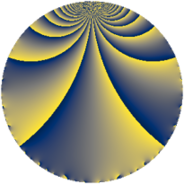# Properties

 Label 2280.2.a.uLevel $2280$ Weight $2$ Character orbit 2280.a Self dual yes Analytic conductor $18.206$ Analytic rank $0$ Dimension $3$ CM no Inner twists $1$

# Related objects

## Newspace parameters

 Level: $$N$$ $$=$$ $$2280 = 2^{3} \cdot 3 \cdot 5 \cdot 19$$ Weight: $$k$$ $$=$$ $$2$$ Character orbit: $$[\chi]$$ $$=$$ 2280.a (trivial)

## Newform invariants

 Self dual: yes Analytic conductor: $$18.2058916609$$ Analytic rank: $$0$$ Dimension: $$3$$ Coefficient field: 3.3.1772.1 Defining polynomial: $$x^{3} - x^{2} - 12 x + 8$$ Coefficient ring: $$\Z[a_1, \ldots, a_{7}]$$ Coefficient ring index: $$2$$ Twist minimal: yes Fricke sign: $$-1$$ Sato-Tate group: $\mathrm{SU}(2)$

## $q$-expansion

Coefficients of the $$q$$-expansion are expressed in terms of a basis $$1,\beta_1,\beta_2$$ for the coefficient ring described below. We also show the integral $$q$$-expansion of the trace form.

 $$f(q)$$ $$=$$ $$q + q^{3} + q^{5} + \beta_{2} q^{7} + q^{9} +O(q^{10})$$ $$q + q^{3} + q^{5} + \beta_{2} q^{7} + q^{9} + ( 2 + \beta_{2} ) q^{11} + ( 1 - \beta_{1} ) q^{13} + q^{15} + 4 q^{17} + q^{19} + \beta_{2} q^{21} + ( -1 + \beta_{1} - \beta_{2} ) q^{23} + q^{25} + q^{27} -\beta_{2} q^{29} -2 q^{31} + ( 2 + \beta_{2} ) q^{33} + \beta_{2} q^{35} + ( -1 + \beta_{1} - 2 \beta_{2} ) q^{37} + ( 1 - \beta_{1} ) q^{39} + ( 1 + \beta_{1} - 2 \beta_{2} ) q^{41} + ( 1 + \beta_{1} ) q^{43} + q^{45} + ( 1 - \beta_{1} + \beta_{2} ) q^{47} + ( 2 - \beta_{1} - \beta_{2} ) q^{49} + 4 q^{51} + ( -3 + \beta_{1} - \beta_{2} ) q^{53} + ( 2 + \beta_{2} ) q^{55} + q^{57} + ( 1 + \beta_{1} - 3 \beta_{2} ) q^{59} + ( 5 + \beta_{1} + \beta_{2} ) q^{61} + \beta_{2} q^{63} + ( 1 - \beta_{1} ) q^{65} + 4 q^{67} + ( -1 + \beta_{1} - \beta_{2} ) q^{69} + ( -2 - 2 \beta_{1} ) q^{71} + 6 q^{73} + q^{75} + ( 9 - \beta_{1} + \beta_{2} ) q^{77} + ( -1 - \beta_{1} - 3 \beta_{2} ) q^{79} + q^{81} + ( -2 - 2 \beta_{2} ) q^{83} + 4 q^{85} -\beta_{2} q^{87} + ( 3 - \beta_{1} + 2 \beta_{2} ) q^{89} + ( -3 - \beta_{1} + 5 \beta_{2} ) q^{91} -2 q^{93} + q^{95} + ( 11 + \beta_{1} - 2 \beta_{2} ) q^{97} + ( 2 + \beta_{2} ) q^{99} +O(q^{100})$$ $$\operatorname{Tr}(f)(q)$$ $$=$$ $$3q + 3q^{3} + 3q^{5} + 3q^{9} + O(q^{10})$$ $$3q + 3q^{3} + 3q^{5} + 3q^{9} + 6q^{11} + 4q^{13} + 3q^{15} + 12q^{17} + 3q^{19} - 4q^{23} + 3q^{25} + 3q^{27} - 6q^{31} + 6q^{33} - 4q^{37} + 4q^{39} + 2q^{41} + 2q^{43} + 3q^{45} + 4q^{47} + 7q^{49} + 12q^{51} - 10q^{53} + 6q^{55} + 3q^{57} + 2q^{59} + 14q^{61} + 4q^{65} + 12q^{67} - 4q^{69} - 4q^{71} + 18q^{73} + 3q^{75} + 28q^{77} - 2q^{79} + 3q^{81} - 6q^{83} + 12q^{85} + 10q^{89} - 8q^{91} - 6q^{93} + 3q^{95} + 32q^{97} + 6q^{99} + O(q^{100})$$

Basis of coefficient ring in terms of a root $$\nu$$ of $$x^{3} - x^{2} - 12 x + 8$$:

 $$\beta_{0}$$ $$=$$ $$1$$ $$\beta_{1}$$ $$=$$ $$($$$$\nu^{2} + 3 \nu - 10$$$$)/2$$ $$\beta_{2}$$ $$=$$ $$($$$$\nu^{2} - \nu - 8$$$$)/2$$
 $$1$$ $$=$$ $$\beta_0$$ $$\nu$$ $$=$$ $$($$$$-\beta_{2} + \beta_{1} + 1$$$$)/2$$ $$\nu^{2}$$ $$=$$ $$($$$$3 \beta_{2} + \beta_{1} + 17$$$$)/2$$

## Embeddings

For each embedding $$\iota_m$$ of the coefficient field, the values $$\iota_m(a_n)$$ are shown below.

For more information on an embedded modular form you can click on its label.

Label $$\iota_m(\nu)$$ $$a_{2}$$ $$a_{3}$$ $$a_{4}$$ $$a_{5}$$ $$a_{6}$$ $$a_{7}$$ $$a_{8}$$ $$a_{9}$$ $$a_{10}$$
1.1
 0.654334 3.67370 −3.32803
0 1.00000 0 1.00000 0 −4.11309 0 1.00000 0
1.2 0 1.00000 0 1.00000 0 0.911179 0 1.00000 0
1.3 0 1.00000 0 1.00000 0 3.20191 0 1.00000 0
 $$n$$: e.g. 2-40 or 990-1000 Significant digits: Format: Complex embeddings Normalized embeddings Satake parameters Satake angles

## Atkin-Lehner signs

$$p$$ Sign
$$2$$ $$1$$
$$3$$ $$-1$$
$$5$$ $$-1$$
$$19$$ $$-1$$

## Inner twists

This newform does not admit any (nontrivial) inner twists.

## Twists

By twisting character orbit
Char Parity Ord Mult Type Twist Min Dim
1.a even 1 1 trivial 2280.2.a.u 3
3.b odd 2 1 6840.2.a.bh 3
4.b odd 2 1 4560.2.a.bs 3

By twisted newform orbit
Twist Min Dim Char Parity Ord Mult Type
2280.2.a.u 3 1.a even 1 1 trivial
4560.2.a.bs 3 4.b odd 2 1
6840.2.a.bh 3 3.b odd 2 1

## Hecke kernels

This newform subspace can be constructed as the intersection of the kernels of the following linear operators acting on $$S_{2}^{\mathrm{new}}(\Gamma_0(2280))$$:

 $$T_{7}^{3} - 14 T_{7} + 12$$ $$T_{11}^{3} - 6 T_{11}^{2} - 2 T_{11} + 32$$ $$T_{13}^{3} - 4 T_{13}^{2} - 38 T_{13} + 164$$

## Hecke characteristic polynomials

$p$ $F_p(T)$
$2$ $$T^{3}$$
$3$ $$( -1 + T )^{3}$$
$5$ $$( -1 + T )^{3}$$
$7$ $$12 - 14 T + T^{3}$$
$11$ $$32 - 2 T - 6 T^{2} + T^{3}$$
$13$ $$164 - 38 T - 4 T^{2} + T^{3}$$
$17$ $$( -4 + T )^{3}$$
$19$ $$( -1 + T )^{3}$$
$23$ $$-32 - 44 T + 4 T^{2} + T^{3}$$
$29$ $$-12 - 14 T + T^{3}$$
$31$ $$( 2 + T )^{3}$$
$37$ $$180 - 78 T + 4 T^{2} + T^{3}$$
$41$ $$344 - 82 T - 2 T^{2} + T^{3}$$
$43$ $$-80 - 42 T - 2 T^{2} + T^{3}$$
$47$ $$32 - 44 T - 4 T^{2} + T^{3}$$
$53$ $$-96 - 16 T + 10 T^{2} + T^{3}$$
$59$ $$688 - 144 T - 2 T^{2} + T^{3}$$
$61$ $$144 - 14 T^{2} + T^{3}$$
$67$ $$( -4 + T )^{3}$$
$71$ $$640 - 168 T + 4 T^{2} + T^{3}$$
$73$ $$( -6 + T )^{3}$$
$79$ $$-1024 - 192 T + 2 T^{2} + T^{3}$$
$83$ $$-200 - 44 T + 6 T^{2} + T^{3}$$
$89$ $$-48 - 50 T - 10 T^{2} + T^{3}$$
$97$ $$-36 + 258 T - 32 T^{2} + T^{3}$$